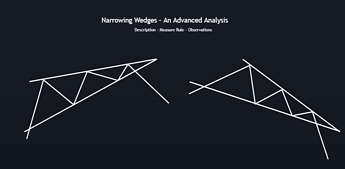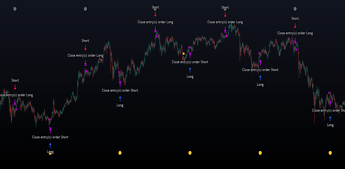# Triangle Patterns - Advanced Analysis

September 22, 2021

Triangle patterns form a part of the most studied patterns by technical analysts and have been well documented over the years, with some even applied to climate time-series data (1). In this post, we perform an analysis of ascending, descending, and symmetrical triangles patterns.

Chart patterns describe distinct structures in financial time series. Their occurrence helps technical analysts predict future price variations.

Triangle patterns form a part of the most studied patterns by technical analysts and have been well documented over the years, with some even applied to climate time-series data (1). In this post, we perform an analysis of ascending, descending, and symmetrical triangles patterns.

We provide a description of each pattern and its implications, as well as a model of the price variation within each described pattern. We also review the literature in order to find their deterministic cause.

To knowledgeable investors, chart patterns are not squiggles on a
price chart; they are the footprints of the smart money.

- Bulkowski (2)

### Ascending Triangles

Ascending triangles are characterized by a series of rising local minima (higher lows) and a series of local maxima staying at a relatively fixed level. A line is drawn from the rising minima, forming an upward sloping support line. Another line is drawn from the maxima, forming a horizontal resistance line. The apex represents the point where both lines intersect.

Ascending Triangles have a bullish bias. Once the price breaks the resistance line we can expect a rapid increase of the price. This breakout is often accompanied by an increase in volume , while the volume prior to the breakout was declining. Note that this is not a pre-requisite.

Example of ascending triangle on CALX daily.

### Descending Triangles

Descending triangles are characterized by a series of declining local maxima (lower highs) and a series of local minima staying at a relatively fixed level. A line is drawn from the declining maxima, forming a downward sloping resistance line. Another line is drawn from the minimal, forming a horizontal support line.

Descending Triangles have a bearish bias. Once the price breaks the support line we can expect a rapid decrease of the price. Like ascending triangles, this breakout is often accompanied by an increase in volume , while the volume prior to the breakout was declining.

Example of descending triangle on CORN daily.

### Symmetrical Triangles

Symmetrical triangles are characterized by a series of declining local maxima (lower highs) and a series of increasing local minima (higher lows). A line is drawn from the declining maxima, forming a downward sloping resistance line. Another line is drawn from the minima, forming an upward sloping support line. Both support and resistance lines should have an approximately equal slope.

Symmetrical triangles do not have a particular bullish or bearish bias, and are sometimes used to indicate market uncertainty. The expected outcomes depend on where a breakout is occurs. If the price breaks the resistance, we can expect an increase of the price, while a breakout of the support can be followed by a decrease of the price.

Example of symmetrical triangle on PFO daily.

### Pattern Modelling

Describing price variations within patterns with a general mathematical formulation can help us describe more complex occurrences of the patterns.

Consider the price within a valid triangle as y'(t), with support S(t) and resistance R(t). We can describe y'(t) as follows:

y'[t] = S[t] + A[t] × (R[t] - S[t]) + e[t]

with A(t) approximately periodic and in an approximate range (0,1) and e(t) as noisy component.

We can see that A(t) is subject to linear damping (the amplitude of price variations within the triangle tend to reduce linearly over time).

This model is very general and can be further developed, but it can be used as the basis for assessing the validity of triangle patterns in the next section.

### Pattern Validity

The validity of a triangle pattern can depend on a wide variety of factors and can change from analyst to analyst.

The price concentration around the support/resistance should be relatively even, that is price should fill the triangle (as described by Bulkowski).

Bulkowski strongly suggests at least two minor highs and two minor lows should be inside the triangle formation. An additional filter is introduced by Bulkowski, the 5% failure, suggesting that a breakout should have a relative distance superior to 5% from the broken line in order to avoid reversals.

Our previous model can be used to determine the validity of a potential triangle pattern . The apex angle is directly related to the magnitude of A(t) and e(t), with lower angle values returning a lower signal to noise ratio. This is bad since A(t) is an essential component for the structure of the triangle. If A(t) ≈ e(t) then we cannot validate the presence of a triangle pattern , since it is more likely to have been the result of noise.

### Measure Rule

The measure rule allows anticipating the magnitude of a breakout. This allows the trader to easily set take profit/stop losses, which enables a higher control over the risk a trader would be taking trading a triangle pattern .

For ascending triangles the predicted magnitude of a breakout is equal to the value of the resistance minus the first local minima inside the triangle.

For descending triangles the predicted magnitude of a breakout is equal to the value of the first local maxima inside the triangle minus the support value.

For symmetrical triangles, the predicted magnitude of a breakout is equal to the highest local maxima inside the triangle minus the lowest local minima inside the triangle.

We can see that for ascending and descending triangles, a breakout of the non-horizontal line would imply a weaker breakout the closer the price is to the apex. In fact, the breakout magnitude would decay linearly. This is also true for symmetrical triangles. This is mentioned by Fisher (3):

- The more the price moves to the very end of a triangle, the weaker will be the breakout in either direction.

### Theoretical Explanation Of The Occurrence Of Triangle Patterns

Explaining the presence of patterns in financial time series is a challenging task. Under a purely efficient market the presence of patterns would simply be the realization of random fluctuations.

A more challenging question would be: "how could market participants cause triangle patterns?"

If we assume that market participants cause the patterns, we know from the pattern descriptions that a mechanism inducing damped oscillatory variations exists. This oscillation is explained by Caginalp and Balenovich by two groups having asymmetric information/opinions (4).

Certain analysts describe triangle patterns as a temporary control switch between sellers and buyers, with scenarios being determined by the amount of energy exhausted by buyers and sellers.

### Conclusion

In this post, we provided a description of triangle patterns. We highlighted the link between the signal-to-noise ratio and the apex angle of a triangle in order to determine its validity, as well as the measure rule for predicting the magnitude of a breakout.

We finally briefly mentioned the theoretical explanation behind the occurrence of triangles patterns in the market. This subject is complex and lacks further research, we highly recommend reading Caginalp & Balevonich on the subject.

Bulkowski offers an extensive number of statistics regarding triangles in his encyclopedia of chart patterns.

### References

(1) Kaiser, J. (2016). Chart Pattern in Climate Time Series Data. Urban & Regional Resilience eJournal.

(2) Bulkowski, T. N. (2021). Encyclopedia of chart patterns. John Wiley & Sons.

(3) Fischer, R., & Fischer, J. (2003). Candlesticks, Fibonacci, and chart pattern trading tools: a synergistic strategy to enhance profits and reduce risk (Vol. 209). John Wiley & Sons.

(4) Caginalp, G., & Balevonich, D. (2003). A Theoretical Foundation for Technical Analysis. Capital Markets: Market Microstructure eJournal.

### Other Stories### Narrowing Wedges - Advanced Analysis

5/13/2022
New Feature
Indicator
Educational

In the last post in this series on chart patterns, we described the characteristics, rules, and causes of broadening wedges patterns (if you haven't seen it, see the related ideas below).### Moon Phases Strategy

5/13/2022
New Feature
Indicator
Educational

Trading moon phases has become quite popular among traders, believing that there exists a relationship between moon phases and market movements. This strategy is based on an estimate of moon phases with the possibility to use different methods to determine long/short positions based on moon phases .